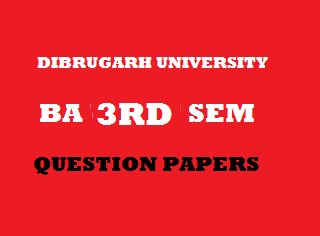## Saturday, January 05, 20192015
(November)
MATHEMATICS
(General)
Course: 301
[Group – A: Coordinate Geometry and
Group – B: Analysis – I (Real Analysis)]
Full Marks: 80
Pass Marks: 32 (Backlog) / 24 (2014 onwards)
Time: 3 hours
The figures in the margin indicate full marks for the questions
GROUP – A
(Coordinate Geometry)
SECTION – I
(2 –Dimension)

1. (a) Write the condition under which the conicis non-singular. 1
(b) Find the new coordinates of the point, if the frame of reference is rotated through an angle, without changing the origin. 2
(c) Find the angle through which it is to be rotated to removeterm from. 2
2. (a)represents a pair of straight lines. Determine whether they are parallel or perpendicular to each other. 1
(b) Find the angle between the pair of lines. 2
(c) Find the pair of lines represented by. 3
(d) Show that the linesandform an equilateral triangle. 6
Or
Discuss the nature of the conic represented by the equationand reduce it to canonical form.
3. (a) Find the equation of tangent atto the conic. 2
(b) Find the centre of the conic. 3
(c) Find the pole of a given line with respect to a conic. 5
Or
Determine whether the conic represented byhas a single centre, infinitely many centres or no centre.

SECTION – II
(3-Dimension)
4. (a) Plane is described by an equation. Write the degree of the equation. 1
(b) The planepasses through a particular point. Write the coordinates of that point. 1
(c) Find the intercepts on the axes made by the plane. 2
(d) Find the equation of the plane passing through the points (3, 1, 1) and (1, -2, 3), and parallel to x-axis. 3
Or
Find the angle between the planesand.
(e) Find the equation of the line in symmetric form passing through (2, 0, 4) and (1, -2, 3). 3
5. (a) Find the length of the shortest distance between the following lines: 4(b) Find the equation of the perpendicular line to the lines;and z-axis.     4

GROUP – B
(Analysis – I)
6. (a) If, then find the value of. 1
(b) If, then find the value of. 2
(c) Find the length of subtangent toat. 3
Or
Find the radius of curvature ofat any point.
(d) Evaluate any one of the following: 4
1.2.7. (a) Write Maclaurin’s theorem with Lagrange’s form of remainder. 1
(b) Write the geometrical meaning of Lagrange’s mean value theorem. 2
(c) Show that a function, which is derivable at a point, is continous at that point. 2
(d) State and prove Rolle’s Theorem. 5
Or
If a functionis derivable on a closed intervaland,are of opposite signs, then there exists at least one pointbetweenandsuch that.
8. (a) If, then find. 1
(b) Verify Euler’s theorem for. 4
Or
If, then show that. 1
9. (a) Write the condition when.
(b) Evaluate any one of the following: 4
1.2.(c) Obtain the reduction formula for. 5
Or

Find the perimeter of the asteroid.